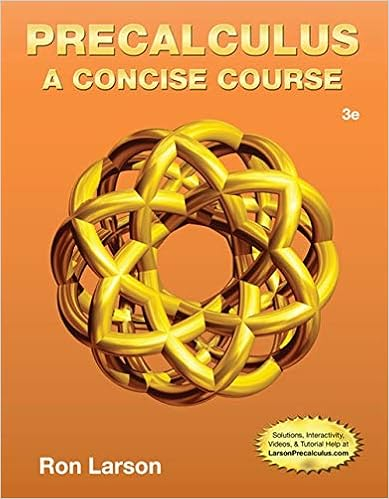# E2 - Exam 2 Principles of Ecology Nov 9 2011 Name This exam...

• Test Prep
• 8

This preview shows page 1 - 2 out of 8 pages.

##### We have textbook solutions for you!
The document you are viewing contains questions related to this textbook.The document you are viewing contains questions related to this textbook.
Chapter 1 / Exercise 49
Precalculus: A Concise Course
LarsonExpert Verified
Exam 2. Principles of Ecology . Nov. 9, 2011. Name ________________________________________ This exam has 100 points with 6 extra credit points possible. I apologize for not asking questions that you knew the answers to! Many important topics have been overlooked. I apologize for this! Here are some useful equations that might be referenced in the exam. N t = N o t N t = N o e rt N t+1 = N t + r o N t (1-N t /K) N t = K/(1 + [(K N o )/N o ] * e rt ) dN/dt = rN(1-N/K) N captured and marked initially = N marked at recapture N total N total recaptured dS/dt = - β SI dS + R dI/dt = β SI vI dI - I dR/dt = vI dR - R dH/dt = rH cHP dP/dt = acHP dP dP/dt = mP(1-P) eP dN 1 /dt = r 1 N 1 (K 1 N 1 12 N 2 )/K 1 dN 2 /dt = r 2 N 2 (K 2 N 2 21 N 1 )/K 2 N t+1 = (s o B + s a ) N t C = L/[S(S-1)/2] Volume sphere = (4/3) pi * r 3 SurfaceArea sphere = 4 * pi * r 2 Multiple guess questions (4 pts each,20 pts total). Answer on this sheet. 1. The population of elk in Yellowstone went from 3300 to 3150 in two years. What was r if the population grew according to the exponential growth during this time? Choose the closest value or write in your vote. a. -0.046 b.  c. 0.05 d.  e. 0.98 f. None of the above. I got __________________ 2. If a population has a spatial dispersion pattern that yields a variance:mean ratio (s 2 /mean) = 0.7 and a X 2 test
yields p = 0.05 then our best interpretation would be that our population dispersion pattern is 3. The food web to the right has a connectance equal to
Principles of Ecology, Exam 2 Page 1 of 8
##### We have textbook solutions for you!
The document you are viewing contains questions related to this textbook.The document you are viewing contains questions related to this textbook.
Chapter 1 / Exercise 49
Precalculus: A Concise Course
LarsonExpert Verified
•••